# GRE Math : How to find an angle in an acute / obtuse isosceles triangle

## Example Questions

### Example Question #1 : How To Find An Angle In An Acute / Obtuse Isosceles Triangle

An isosceles triangle has an angle of 110°.  Which of the following angles could also be in the triangle?

35

90

110

20

55

35

Explanation:

An isosceles triangle always has two equal angles. As there cannot be another 110° (the triangle cannot have over 180° total), the other two angles must equal eachother. 180° - 110° = 70°. 70° represents the other two angles, so it needs to be divided in 2 to get the answer of 35°.

### Example Question #2 : How To Find An Angle In An Acute / Obtuse Isosceles Triangle

An isosceles triangle ABC is laid flat on its base.  Given that <B, located in the lower left corner, is 84 degrees, what is the measurement of the top angle, <A?

96
42
84
12
20
Explanation:

Since the triangle is isosceles, and <A is located at the top of the triangle that is on its base, <B and <C are equivalent.  Since <B is 84 degrees, <C is also.  There are 180 degrees in a triangle so 180 - 84 - 84 = 12 degrees.

### Example Question #3 : How To Find An Angle In An Acute / Obtuse Isosceles Triangle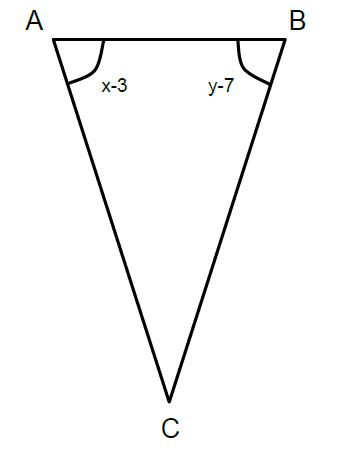Triangle ABC is isosceles

x and y are positive integers

A

---

x

B

---

y

The two quantities are equal

The relationship cannot be determined

Quantity B is greater

Quantity A is greater

Quantity B is greater

Explanation:

Since we are given expressions for the two congruent angles of the isosceles triangle, we can set the expressions equal to see how x relates to y. We get,

x – 3 = y – 7 --> y = x + 4

Logically, y must be the greater number if it takes x an additional 4 units to reach its value (knowing they are both positive integers).

### Example Question #4 : How To Find An Angle In An Acute / Obtuse Isosceles Triangle

An isosceles triangle has one obtuse angle that is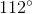. What is the value of one of the other angles?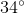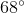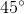Explanation:

We know that an isosceles triangel has two equal sides and thus two equal angles opposite those equal sides. Because there is one obtuse angle of 112 degrees we automatically know that this angle is the vertex. If you sum any triangle's interior angles, you always get 180 degrees.

180 – 112 = 68 degrees. Thus there are 68 degrees left for the two equal angles. Each angle must therefore measure 34 degrees.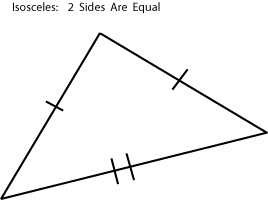Tired of practice problems?

Try live online GRE prep today.